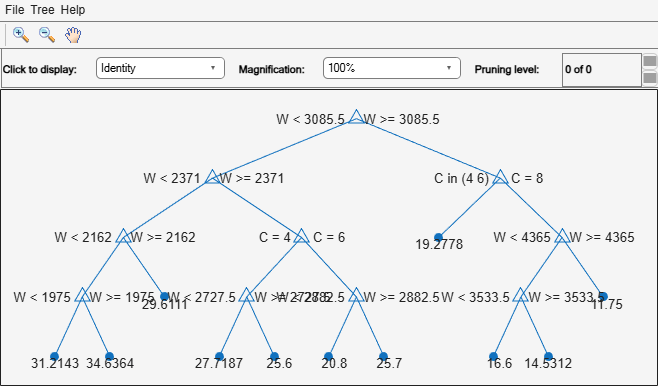# CompactRegressionEnsemble

Package: classreg.learning.regr

Compact regression ensemble class

## Description

Compact version of a regression ensemble (of class `RegressionEnsemble`). The compact version does not include the data for training the regression ensemble. Therefore, you cannot perform some tasks with a compact regression ensemble, such as cross validation. Use a compact regression ensemble for making predictions (regressions) of new data.

## Construction

```ens = compact(fullEns)``` constructs a compact decision ensemble from a full decision ensemble.

### Input Arguments

 `fullEns` A regression ensemble created by `fitrensemble`.

## Properties

 `CategoricalPredictors` Categorical predictor indices, specified as a vector of positive integers. `CategoricalPredictors` contains index values corresponding to the columns of the predictor data that contain categorical predictors. If none of the predictors are categorical, then this property is empty (`[]`). `CombineWeights` A character vector describing how the ensemble combines learner predictions. `ExpandedPredictorNames` Expanded predictor names, stored as a cell array of character vectors. If the model uses encoding for categorical variables, then `ExpandedPredictorNames` includes the names that describe the expanded variables. Otherwise, `ExpandedPredictorNames` is the same as `PredictorNames`. `NumTrained` Number of trained learners in the ensemble, a positive scalar. `PredictorNames ` A cell array of names for the predictor variables, in the order in which they appear in `X`. `ResponseName` A character vector with the name of the response variable `Y`. `ResponseTransform` Function handle for transforming scores, or character vector representing a built-in transformation function. `'none'` means no transformation; equivalently, `'none'` means `@(x)x`. Add or change a `ResponseTransform` function using dot notation: `ens.ResponseTransform = @function` `Trained` The trained learners, a cell array of compact regression models. `TrainedWeights` A numeric vector of weights the ensemble assigns to its learners. The ensemble computes predicted response by aggregating weighted predictions from its learners.

## Object Functions

 `loss` Regression error `partialDependence` Compute partial dependence `plotPartialDependence` Create partial dependence plot (PDP) and individual conditional expectation (ICE) plots `predict` Predict responses using ensemble of regression models `predictorImportance` Estimates of predictor importance for regression ensemble `removeLearners` Remove members of compact regression ensemble

## Copy Semantics

Value. To learn how value classes affect copy operations, see Copying Objects.

## Examples

collapse all

Create a compact regression ensemble for efficiently making predictions on new data.

Load the `carsmall` data set. Consider a model that explains a car's fuel economy (`MPG`) using its weight (`Weight`) and number of cylinders (`Cylinders`).

```load carsmall X = [Weight Cylinders]; Y = MPG;```

Train a boosted ensemble of 100 regression trees using the `LSBoost`. Specify that `Cylinders` is a categorical variable.

```Mdl = fitrensemble(X,Y,'PredictorNames',{'W','C'},... 'CategoricalPredictors',2)```
```Mdl = RegressionEnsemble PredictorNames: {'W' 'C'} ResponseName: 'Y' CategoricalPredictors: 2 ResponseTransform: 'none' NumObservations: 94 NumTrained: 100 Method: 'LSBoost' LearnerNames: {'Tree'} ReasonForTermination: 'Terminated normally after completing the requested number of training cycles.' FitInfo: [100x1 double] FitInfoDescription: {2x1 cell} Regularization: [] Properties, Methods ```

`Mdl` is a `RegressionEnsemble` model object that contains the training data, among other things.

Create a compact version of `Mdl`.

`CMdl = compact(Mdl)`
```CMdl = CompactRegressionEnsemble PredictorNames: {'W' 'C'} ResponseName: 'Y' CategoricalPredictors: 2 ResponseTransform: 'none' NumTrained: 100 Properties, Methods ```

`CMdl` is a `CompactRegressionEnsemble` model object. `CMdl` is almost the same as `Mdl`. One exception is that `CMdl` does not store the training data.

Compare the amounts of space consumed by `Mdl` and `CMdl`.

```mdlInfo = whos('Mdl'); cMdlInfo = whos('CMdl'); [mdlInfo.bytes cMdlInfo.bytes]```
```ans = 1×2 514407 489348 ```

`Mdl` consumes more space than `CMdl`.

`CMdl.Trained` stores the trained regression trees (`CompactRegresionTree` model objects) that compose `Mdl`.

Display a graph of the first tree in the compact ensemble.

`view(CMdl.Trained{1},'Mode','graph');`By default, `fitrensemble` grows shallow trees for boosted ensembles of trees.

Predict the fuel economy of a typical car using the compact ensemble.

```typicalX = [mean(X(:,1)) mode(X(:,2))]; predMeanX = predict(CMdl,typicalX)```
```predMeanX = 26.2520 ```

## Tips

For a compact ensemble of regression trees, the `Trained` property of `ens` stores a cell vector of `ens.NumTrained` `CompactRegressionTree` model objects. For a textual or graphical display of tree `t` in the cell vector, enter

`view(ens.Trained{t})`